Test: Quantitative Techniques- 7

# Test: Quantitative Techniques- 7

Test Description

## 20 Questions MCQ Test IBPS PO Prelims- Study Material, Online Tests, Previous Year | Test: Quantitative Techniques- 7

Test: Quantitative Techniques- 7 for Banking Exams 2023 is part of IBPS PO Prelims- Study Material, Online Tests, Previous Year preparation. The Test: Quantitative Techniques- 7 questions and answers have been prepared according to the Banking Exams exam syllabus.The Test: Quantitative Techniques- 7 MCQs are made for Banking Exams 2023 Exam. Find important definitions, questions, notes, meanings, examples, exercises, MCQs and online tests for Test: Quantitative Techniques- 7 below.
Solutions of Test: Quantitative Techniques- 7 questions in English are available as part of our IBPS PO Prelims- Study Material, Online Tests, Previous Year for Banking Exams & Test: Quantitative Techniques- 7 solutions in Hindi for IBPS PO Prelims- Study Material, Online Tests, Previous Year course. Download more important topics, notes, lectures and mock test series for Banking Exams Exam by signing up for free. Attempt Test: Quantitative Techniques- 7 | 20 questions in 30 minutes | Mock test for Banking Exams preparation | Free important questions MCQ to study IBPS PO Prelims- Study Material, Online Tests, Previous Year for Banking Exams Exam | Download free PDF with solutions
 1 Crore+ students have signed up on EduRev. Have you?
Test: Quantitative Techniques- 7 - Question 1

### If a man sells two articles at Rs125 each. On one he gains 10% while on other he loses 10%. What is the gain or loss percentage on whole transaction?

Detailed Solution for Test: Quantitative Techniques- 7 - Question 1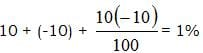So Loss 1%

Test: Quantitative Techniques- 7 - Question 2

### A train takes 5 sec to pass an electric pole. If the length of the train is 120 m, then the time taken by it to cross a railway platform 180 m long is

Detailed Solution for Test: Quantitative Techniques- 7 - Question 2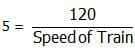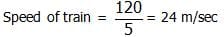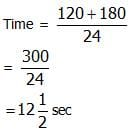Test: Quantitative Techniques- 7 - Question 3

### If I walk from my house to my office at 5kmph, I reach late by 30 mins but if I walk at 6kmph I am late by 5 mins only. Find the distance from my office to my house?

Detailed Solution for Test: Quantitative Techniques- 7 - Question 3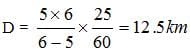Test: Quantitative Techniques- 7 - Question 4

A, B, C hired a car for Rs. 520 and used it for 7, 8 and 11 hours respectively. Hire charges paid by B were:

Detailed Solution for Test: Quantitative Techniques- 7 - Question 4

A : B : C = 7 : 8 : 11.
Hire chares paid by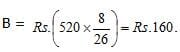Test: Quantitative Techniques- 7 - Question 5

A is 75% of B. Then ratio change of B to A must be?

Detailed Solution for Test: Quantitative Techniques- 7 - Question 5

A : B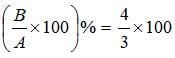= 133.33%

Test: Quantitative Techniques- 7 - Question 6

If diagonal of the rectangle is 17cm long and it’s perimeter is 46cm, find the area of the rectangle

Detailed Solution for Test: Quantitative Techniques- 7 - Question 6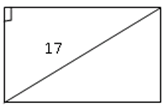2(L+B) = 46
L + B = 23
So, L 15, B = 8, D = 17
Area = 18 x 8 = 120

Test: Quantitative Techniques- 7 - Question 7

A can do a work in 12 days. When he had worked for 3 days, B joined him. If they complete the work in 3 more days, in how many days can B alone finish the work?

Detailed Solution for Test: Quantitative Techniques- 7 - Question 7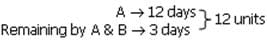A's one day work = 1 units
A's 3 day's work = 3 units
Remaining work = 9 units
One day work of A & B = 3 units
So, B's one day work = 3 - 1 = 2
B will complete whole work in = 12/2 = 6days

Test: Quantitative Techniques- 7 - Question 8

A person found average of 10 numerals as 5. After scruting he found that he made mistakes at 2 places and wrongly read as 15 instead of 45 and 30 instead of 10. What is the correct average?

Detailed Solution for Test: Quantitative Techniques- 7 - Question 8

Correct average =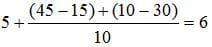Test: Quantitative Techniques- 7 - Question 9

If the sum of two numbers are 33 and their difference is 13. Find their product?

Detailed Solution for Test: Quantitative Techniques- 7 - Question 9

a + b = 33 2a = 46
a - b = 13
a = 23 a x b = 23 x 10 = 230
b = 10

Test: Quantitative Techniques- 7 - Question 10

Mohit’s age after 6 year will be 3/7 of his father’s age. Ten years ago the ratio of their ages was 1:5. What is Mohit’s father’s age at present?

Detailed Solution for Test: Quantitative Techniques- 7 - Question 10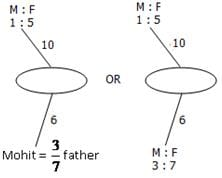So 2 ≡ 16 yrs
1 ≡ 8 yrs
5 ≡ 40 yrs
Age of father 10 years ago = 40 yrs
Present age of father = 40 + 10 = 50 yrs

Test: Quantitative Techniques- 7 - Question 11

The ratio between present ages of P and Q is 6:7. If Q is 4 years older than P, what will be the ratio of their ages after 4 years?

Detailed Solution for Test: Quantitative Techniques- 7 - Question 11

P : Q  Diff  So, P = 24
6 : 7  x 4       Q = 28
So after 4 years 28, 32
i.e ratio will be  7 : 8

Test: Quantitative Techniques- 7 - Question 12

Two numbers are less than third number by 30% and 37% respectively. How much % in the second number less than first number?

Detailed Solution for Test: Quantitative Techniques- 7 - Question 12

A  B  C
70  63  100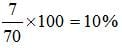Test: Quantitative Techniques- 7 - Question 13

The students in three classes are in the ratio 2 : 3 : 5. If 40 students are increased in each class, the ratio changes to 4 : 5 : 7. Originally, the total number of students was

Detailed Solution for Test: Quantitative Techniques- 7 - Question 13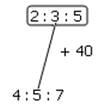So 2 ≡ 40
and 1 ≡ 20
So total no. of students = 10 x 20 = 200.

Test: Quantitative Techniques- 7 - Question 14

Monthly income of P and Q are in the ratio 2:3 and the monthly expenses were in the ratio 5:9. If each of them saves 300 per month, find their respective incomes?

Detailed Solution for Test: Quantitative Techniques- 7 - Question 14

2x -5y = 300
3x – 9y = 300
Income = 800, 1200

Test: Quantitative Techniques- 7 - Question 15

A and B invested in the ratio of 2:3 respectively. If A had invested Rs 10000 more, the ratio of A investment to B would have been 3:2. What was the amount invested by A?

Detailed Solution for Test: Quantitative Techniques- 7 - Question 15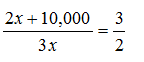4x + 20,000
5x = 20,000
x = 4000
So, A invested 8000

Test: Quantitative Techniques- 7 - Question 16

A bike crosses a bridge at a speed of 180kmph. What will be length of bridge if bike takes 3 mins to cross the bridge?

Detailed Solution for Test: Quantitative Techniques- 7 - Question 16

S = 180 Km/h
D = ?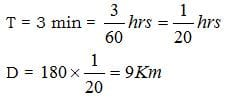Test: Quantitative Techniques- 7 - Question 17

15 men, 18 women and 12 boys working together earns Rs. 2070. If daily wages of a man, a woman and a boy are in the ratio 4:3:2. The daily wage of 1 man and 2 women and 3 boys are?

Detailed Solution for Test: Quantitative Techniques- 7 - Question 17

: (15 x 4) : (18 x 3) : (12 x 2)
60: 54 + 24 → Sum 2070
138 ≡ 2070
1 ≡ 15
So, [(1 x 4) + (2 x 3) + (3 x 2)] x 15
= 16 x 15 = 240

Test: Quantitative Techniques- 7 - Question 18

The difference between simple and compound interest on a certain sum of money at 5% rate of interest for 2 years is Rs. 15. The sum is?

Detailed Solution for Test: Quantitative Techniques- 7 - Question 18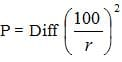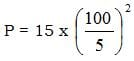= 15 x 400 = 6000

Test: Quantitative Techniques- 7 - Question 19

A boat goes at a speed of 11km/hr downstream and 5km/hr upstream in 7 hours. Speed of stream (in km/hr)?

Detailed Solution for Test: Quantitative Techniques- 7 - Question 19

Speed of water =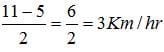Test: Quantitative Techniques- 7 - Question 20

Three numbers are in the ratio 3:4:5 and their LCM is 2400.their HCF is?

Detailed Solution for Test: Quantitative Techniques- 7 - Question 20

Let nos. = 3x, 4x, 5x  60x ≡ 2400
So (3 x 40), (4 x 40), (5 x 40)
H.C.F = 40

## IBPS PO Prelims- Study Material, Online Tests, Previous Year

173 videos|192 docs|149 tests
 Use Code STAYHOME200 and get INR 200 additional OFF Use Coupon Code
Information about Test: Quantitative Techniques- 7 Page
In this test you can find the Exam questions for Test: Quantitative Techniques- 7 solved & explained in the simplest way possible. Besides giving Questions and answers for Test: Quantitative Techniques- 7, EduRev gives you an ample number of Online tests for practice

## IBPS PO Prelims- Study Material, Online Tests, Previous Year

173 videos|192 docs|149 tests